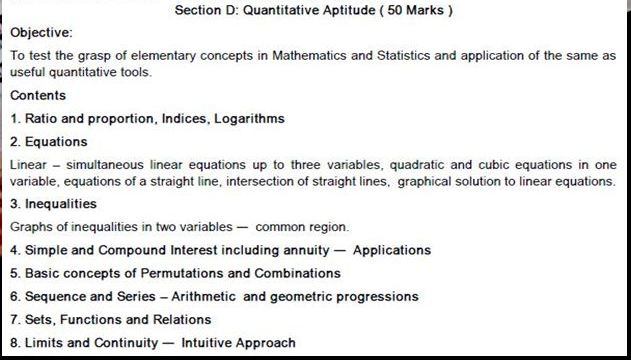# CA CS ICWA KNOWLEDGE HUB - STUDENTS - CPT QUANTITATIVE APTITUDE STUDY MATERIAL , LECTURE SERIES, ONLINE SELF ASSESSMENT QUIZ

CA CS ICWA KNOWLEDGE HUB - STUDENTS

STUDY MATERIAL

QUANTITATIVE APTITUDE

This Quiz consists of 25 questions to be answered in 30 Minutes with a passing requirement of 50%

SELF ASSESSMENT QUIZ - CHAPTER WISE
This Quiz consists of 12 questions to be answered 15 minutes with a passing requirement of 50%.

 Chapter-1 Ratio and Proportion,indices and logarithms Chapter-2 Equations Chapter-3 Inequalities Chapter-4 Simple and Compound interest Including Annuity Applications Chapter-5 Basic Concepts of Permutations and Combinations Chapter-6 Sequence and series-Arithmetic and Geometric Progression  Chapter-7 Sets ,Functions and Relations Chapter-8 Limits and Continuity-Intutive Approach Chapter-9 Basic Concepts of Differential and Integral Calculus Chapter-10 Statistical Description of Data Chapter-11 Measures of Central Tendency and Dispersion Chapter-12 Correlation and Regression Chapter-13 Probability and Expected value by Mathematical Expectation Chapter-14 Theretical Distributions Chapter-15 Sampling Theory Chapter-16 Index numbers                                                                       SYLLABUS